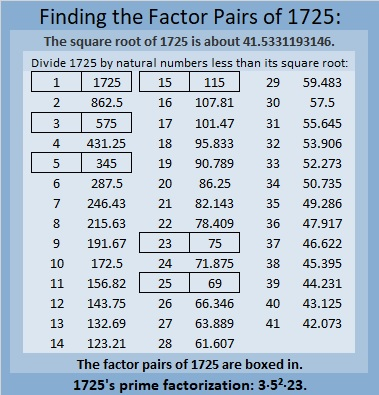# 1725 A Toast to You on This Last Day of the Year

### Today’s Puzzle:

I haven’t posted as much this past year as I ought, but thank you for bearing with me. Here is a toast to you, faithful reader! Here’s hoping for a great new year for all of us! Write the numbers from 1 to 10 in both the first column and the top row so that the given clues are the products of the factors you write.### Factors of 1725:

• 1725 is a composite number.
• Prime factorization: 1725 = 3 × 5 × 5 × 23, which can be written 1725 = 3 × 5² × 23.
• 1725 has at least one exponent greater than 1 in its prime factorization so √1725 can be simplified. Taking the factor pair from the factor pair table below with the largest square number factor, we get √1725 = (√25)(√69) = 5√69.
• The exponents in the prime factorization are 1, 2, and 1. Adding one to each exponent and multiplying we get (1 + 1)(2 + 1)(1 + 1) = 2 × 3 × 2 = 12. Therefore 1725 has exactly 12 factors.
• The factors of 1725 are outlined with their factor pair partners in the graphic below.### More About the Number 1725:

1725 is the difference of two squares in six different ways:

863² – 862² = 1725,
289² – 286² = 1725,
175² – 170² = 1725,
65² – 50² = 1725,
49² – 26² = 1725, and
47² – 22² = 1725.